• 快速傅里叶变换原理及其应用,很不错的选择！算法很详细说明！ 快速傅里叶变换原理及其应用,很不错的选择！算法很详细说明！ 快速傅里叶变换原理及其应用,很不错的选择！算法很详细说明！ 快速傅里叶变换原理及其应用...
• 傅里叶变换、离散傅里叶变换、快速傅里叶变换的介绍
• 本文主要讲解图像傅里叶变换的相关内容，在数字图像处理中，有两个经典的变换被广泛应用——傅里叶变换和霍夫变换。其中，傅里叶变换主要是将时间域上的信号转变为频率域上的信号，用来进行图像除噪、图像增强等处理...
该系列文章是讲解Python OpenCV图像处理知识，前期主要讲解图像入门、OpenCV基础用法，中期讲解图像处理的各种算法，包括图像锐化算子、图像增强技术、图像分割等，后期结合深度学习研究图像识别、图像分类应用。希望文章对您有所帮助，如果有不足之处，还请海涵~
前面一篇文章我讲解了Python图像量化、采样处理及图像金字塔。本文主要讲解图像傅里叶变换的相关内容，在数字图像处理中，有两个经典的变换被广泛应用——傅里叶变换和霍夫变换。其中，傅里叶变换主要是将时间域上的信号转变为频率域上的信号，用来进行图像除噪、图像增强等处理。基础性文章，希望对你有所帮助。 同时，该部分知识均为杨秀璋查阅资料撰写，未经授权禁止转载，谢谢！！如果有问题随时私聊我，只望您能从这个系列中学到知识，一起加油喔~
1.图像傅里叶变换 2.Numpy实现傅里叶变换 3.Numpy实现傅里叶逆变换 4.OpenCV实现傅里叶变换 5.OpenCV实现傅里叶逆变换
该系列在github所有源代码：

https://github.com/eastmountyxz/ImageProcessing-Python

PS：请求帮忙点个Star，哈哈，第一次使用Github，以后会分享更多代码，一起加油。
同时推荐作者的C++图像系列知识：
展开全文Python图像处理 OpenCV
• 数字图像处理-傅里叶变换马殿富 dfma@nlsde.buaa.edu.cn 2002.9.29 变换问题的引入 数字图像正交变换 傅里叶变换 沃尔什变换 哈达玛变换 离散余弦变换 K-L变换 小波变换 傅里叶变换 傅里叶变换是线性系统分析的一个...

数字图像处理-傅里叶变换
马殿富 dfma@nlsde.buaa.edu.cn 2002.9.29 变换问题的引入 数字图像正交变换 傅里叶变换 沃尔什变换 哈达玛变换 离散余弦变换 K-L变换 小波变换 傅里叶变换 傅里叶变换是线性系统分析的一个有力工具，它能够定量地分析诸如数字图像之类的数字化系统，把傅里叶变换的理论与物理解释相结合，将有利于解决大多数图像处理问题。 幅值 频率 一维基函数 一维傅立叶变换定义 设 x(n):x(0),x(1),……,x(N-1); X(m): X(0), X(1),……,X(N-1)是数字序列， 则序列x(n)的傅立叶变换生成序列X(m)表示如下： x(n)是输入函数，X(m)是输出函数，N=8 二维傅立叶变换定义 图像矩阵 实数 二维傅立叶变换定义 设 f(x,y):f(0,0),f(0,1),……,f(0,N-1), f(1,0),f(1,1),……,f(1,N-1), ……. f(N-1,0),f(N-1,1),……,f(N-1,N-1),是数字矩阵 F(u,v): f(x,y):f(0,0),f(0,1),……,f(0,N-1), f(1,0),f(1,1),……,f(1,N-1), ……. f(N-1,0),f(N-1,1),……,f(N-1,N-1),是数字矩阵 则f(x,y)的傅立叶变换生成F(u,v)表示如下： 二维傅立叶变换 傅立叶变换：F(u,v)=|F(u,v)|ej?(u,v) 傅立叶谱： |F(u,v)|= [R2(u,v)+I2(u,v)]1/2 相位 ?(u,v)=arctan(I(u,v)/R(u,v)) 能量谱: E=|F(u,v)|2 二维傅立叶变换 二维傅立叶变换示例(1) 二维傅立叶变换示例(2) 傅里叶变换示例 傅立叶变换示例(1) 图像中的周期性噪声产生了变换中的尖峰信号 幅值与相位 傅立叶变换示例(2.1) 傅里叶变换性质1 可分离性 二维傅里叶变换可以分离为一维傅里叶变换处理 傅里叶变换性质1 可分离性 图像 傅立叶变换性质2 周期性 如果f(x,y)?F(u,v),则 傅立叶变换性质3 平移性 傅立叶变换性质3 平移性 如果f(x,y)?F(u,v),则 f(x,y)exp[j2?(u0x+v0y)/N]?F(u-u0,v-v0) f(x-x0,y-y0)?F(u,v)exp[-j2?(ux0+vy0)/N] 傅立叶变换性质4 共轭对称性 如果f(x,y)?F(u,v), F*(-u,-v)是共轭复数，则 F(u,v)= F*(-u,-v) |F(u,v)|= |F*(-u,-v)| 傅立叶变换性质5 旋转 傅立叶变换性质5 旋转 设f(x,y)?F(u,v), 傅立叶变换性质6 线性 如果f1(x,y)?F1(u,v), f2(x,y)?F2(u,v),则 傅立叶变换性质7 比例性 如果f(x,y)?F(u,v),则 傅立叶变换性质7 平均值 傅立叶变换性质拉普拉斯算子 如果f(x,y)?F(u,v),并且 ▽2f(x,y)=?2f(x,y)/?x2+?2f(x,y)/?y2,则 ▽2f(x,y)= -(2?)2(u2+v2)F(u,v) 卷积定义 一维卷积定义： 卷积示例(1.1) f(x)和g(x)作卷积 卷积示例(1.2) f(?)和 g(x- ?) 乘积 卷积示例(2) 函数f(x) 卷积示例(3) 折叠 卷积定理 如果f(x,y)?F(u,v), g(x,y) ? G(u,v) 则f(x,y)*g(x,y) ? F(u,v)G(u,v) 许多图像变换是卷积运算 在频域的乘积运算比在空域的卷积运算快，特别是有了快速傅立叶变换以后，效果更加明显。 相关定义 一维相关定义： 相关定理 如果f(x,y)?F(u,v), g(x,y) ? G(u,v) 则f(x,y)?g(x,y) ? F(u,v)G*(u,v) 相关主要应用于模板和原型匹配 给定一个未知图像和已知图像集之间求最紧密的匹配。其基本途径是求相关，然后取相关函数最大值。 能量(Rayleigh)定理 能量定义 能量定理 快速傅立叶变换(1) 1850年，高斯给出了DFT有效算法 1942年，丹尼尔森证明了一个界长为N的傅立叶变换可以由两个界长为N/2的傅立叶变换表示。 1964年，库利-图基给出了快速傅立叶变换算法 快速傅立叶变换(1) 计算复杂性：N乘法，N(N-1)加法 快速傅立叶变换(2) W是周期为N的周期函数 W=cos (2?/N) -

展开全文• 傅里叶变换 冷建华编著 清华大学出版 主要讲解傅里叶变换原理及应用
• 本文由浅入深讲解了傅里叶变换原理，非常适合初学者和有一定基础的的人员。在理论讲解完成之后，还利用在数字图像处理、人脸识别等实际应用的例子，更加具体的对傅里叶变换应用场景进行了详细的描述。是关于...
• 本专栏主要介绍如果通过OpenCv-Python进行图像处理，通过原理理解OpenCv-Python的函数处理原型，在具体情况中，针对不同的图像进行不同等级的、不同方法的处理，以达到对图像进行去噪、锐化等一系列的操作。...
本专栏主要介绍如果通过OpenCv-Python进行图像处理，通过原理理解OpenCv-Python的函数处理原型，在具体情况中，针对不同的图像进行不同等级的、不同方法的处理，以达到对图像进行去噪、锐化等一系列的操作。同时，希望观看本专栏的小伙伴可以理解到OpenCv进行图像处理的强大哦，如有转载，请注明出处(原文链接和作者署名)，感谢各位小伙伴啦！
前文参考： 《OpenCv视觉之眼》Python图像处理一 :Opencv-python的简介及Python环境搭建 《OpenCv视觉之眼》Python图像处理二 :Opencv图像读取、显示、保存基本函数原型及使用 《OpenCv视觉之眼》Python图像处理三 :Opencv图像属性、ROI区域获取及通道处理 《OpenCv视觉之眼》Python图像处理四 :Opencv图像灰度处理的四种方法及原理 《OpenCv视觉之眼》Python图像处理五 :Opencv图像去噪处理之均值滤波、方框滤波、中值滤波和高斯滤波
上次博客，我们讲解了OpenCV图像去噪的几种基本方法，包括均值滤波、中值滤波、高斯滤波等，也就是在图像时域上进行去噪的方法，其中，高斯滤波和中值滤波是需要重点掌握的图像去噪方法，因为对图像的高斯噪声和椒盐噪声去除的效果比较好；除了以上时域上的去噪方法之外，OpenCV也提供了对图像频域上的去噪的函数方法，例如高通滤波和低通滤波，通常用作图像除噪、图像增强和锐化等，因此，高通滤波和低通滤波也是我们学习OpenCV需要掌握的两种图像去噪的方法，但由于高通和低通滤波的实现原理是通过傅里叶变换后进行处理，然后通过傅里叶逆变换进行实现的，所以首先我们需要了解图像傅里叶变换和傅里叶逆变换的原理。
本次博客，林君学长将带大家了解OpenCV图像的傅里叶变换和逆变换原理，通过原理编写傅里叶变换和逆变换的功能函数，并同时介绍OpenCV傅里叶变换和逆变换的库函数使用方法，一起学习吧！
在进行傅里叶变换学习时，我们必须要明白为什么要在频率域研究图像增强？
可以利用频率成分和图像外表之间的对应关系。一些在空间域表述困难的增强任务，在频率域中变得非常普通滤波在频率域更为直观，它可以解释空间域滤波的某些性质可以在频率域指定滤波器，做反变换，然后在空间域使用结果滤波器作为空间域滤波器的指导一旦通过频率域试验选择了空间滤波，通常实施都在空间域进行
提示：傅里叶变换是为后面低通滤波或者说是高通滤波做准备哦，一般来说，处理的是灰度图像，通过上面的介绍我们也可以看出，但下面的原理我们通过处理彩色图像编写的，而OpenCV库函数是在处理灰度图像基础上实现的

[Python图像处理六] :Opencv图像傅里叶变换和傅里叶逆变换原理及实现
一、傅里叶变换1、傅里叶变换原理2、自定义傅里叶变换功能函数3、OpenCV库函数实现傅里叶变换
二、傅里叶逆变换1、傅里叶逆变换原理2、自定义傅里叶逆变换功能函数3、OpenCV库函数实现傅里叶逆变换

一、傅里叶变换
傅里叶变换（Fourier Transform，FT）后，对同一事物的观看角度随之改变，可以从频域里发现一些从时域里不易察觉的特征。某些在时域内不好处理的地方，在频域内可以容易地处理。
1、傅里叶变换原理
1）、什么是傅里叶变换？ 能将满足一定条件的某个函数表示成三角函数（正弦和/或余弦函数）或者它们的积分的线性组合。在不同的研究领域，傅立叶变换具有多种不同的变体形式，如连续傅立叶变换和离散傅立叶变换。傅里叶变换是一种分析信号的方法，它可分析信号的成分，也可用这些成分合成信号。许多波形可作为信号的成分，比如正弦波、方波、锯齿波等，傅里叶变换用正弦波作为信号的成分。 傅里叶变换的实质是将一个信号分离为无穷多多正弦/复指数信号的加成，也就是说，把信号变成正弦信号相加的形式——既然是无穷多个信号相加，那对于非周期信号来说，每个信号的加权应该都是零——但有密度上的差别，你可以对比概率论中的概率密度来思考一下——落到每一个点的概率都是无限小，但这些无限小是有差别的所以，傅里叶变换之后，横坐标即为分离出的正弦信号的频率，纵坐标对应的是加权密度。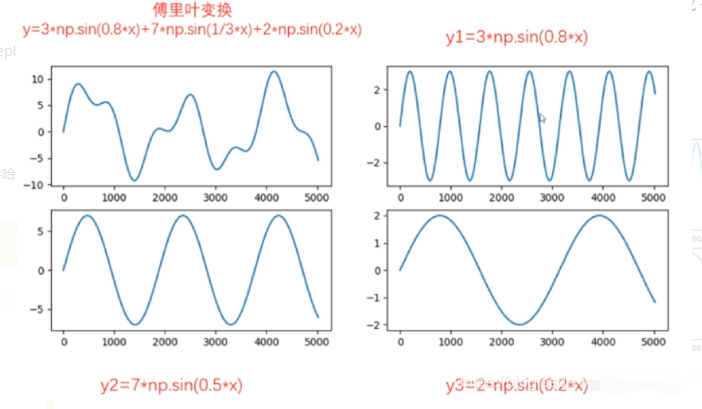2）、傅里叶变换作用？ 傅里叶变换可以将一个时域信号转换成在不同频率下对应的振幅及相位，其频谱就是时域信号在频域下的表现，而反傅里叶变换可以将频谱再转换回时域的信号。最简单最直接的应用就是时频域转换。将图像时域处理上面复杂的特征转为频域实现简单化，对简单化的特征进行处理，然后再通过傅里叶逆变换回到原来的图像，如下所示：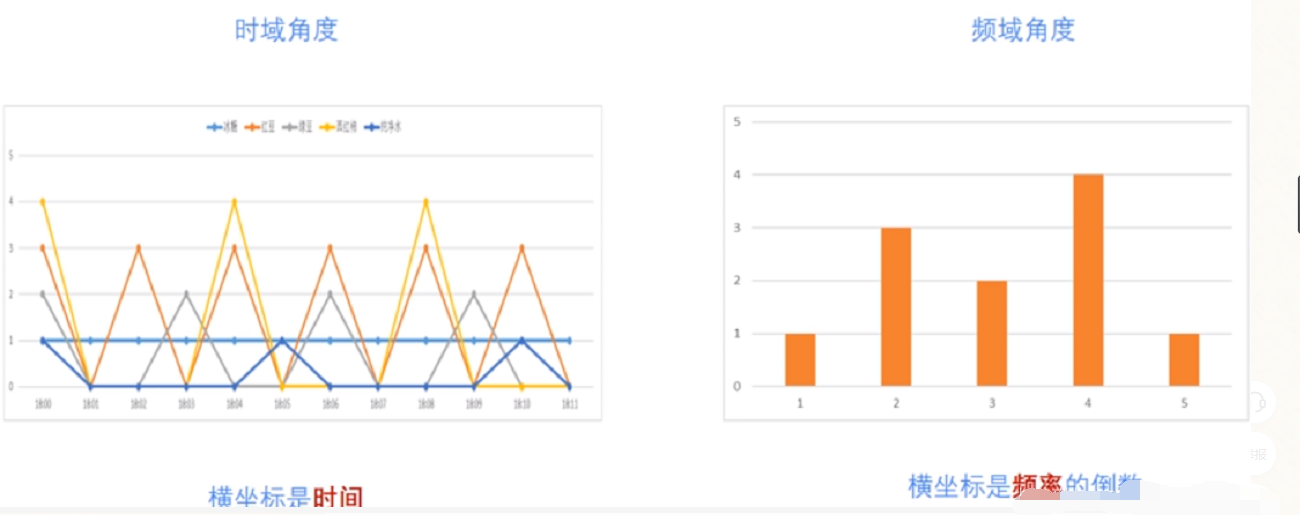3）、图像进行傅里叶变换原理 我们知道，灰度图像是由二维的离散的点构成的。二维离散傅里叶变换（Two-Dimensional Discrete Fourier Transform）常用于图像处理中，对图像进行傅里叶变换后得到其频谱图。频谱图中频率高低表征图像中灰度变化的剧烈程度。图像中边缘和噪声往往是高频信号，而图像背景往往是低频信号。我们在频率域内可以很方便地对图像的高频或低频信息进行操作，完成图像去噪，图像增强，图像边缘提取等操作，因此，对图像进行傅里叶变换的原理如下公式：

F

(

u

,

v

)

=

∑

x

=

0

H

−

1

∑

y

=

0

W

−

1

f

(

x

,

y

)

e

−

2

j

π

(

u

x

/

H

+

v

y

/

W

)

图

像

高

H

，

宽

W

。

F

(

u

,

v

)

表

示

频

域

图

像

，

f

(

x

,

y

)

表

示

时

域

图

像

。

u

的

范

围

为

[

0

,

H

−

1

]

，

v

的

范

围

为

[

0

,

W

−

1

]

↑

F(u,v)=\sum_{x=0}^{H-1}\sum_{y=0}^{W-1}f(x,y)e^{-2j\pi(ux/H+vy/W)} \\\\ 图像高H，宽W。F(u,v)表示频域图像，f(x,y)表示时域图像。u的范围为[0,H-1]，v的范围为[0,W-1] ↑

那么怎么将上面的二维公式转为我们可以理解的二维图像呢？其实很简单，

f

(

x

,

y

)

f(x,y)

表示在(x，y)点上的图像的像素值，该像素值乘以后面的在(u,v)点的

e

−

2

j

π

(

u

x

/

H

+

v

y

/

W

)

e^{-2j\pi(ux/H+vy/W)}

处理出来的一个数，然后对图像所有像素进行遍历，最后求和，就得到在频域上该(u,v)点对应的值，通过该原理，我们就可以自己写出傅里叶变换的功能函数了，来看吧
2、自定义傅里叶变换功能函数
1）、自定义傅里叶变换函数
import cv2
import numpy as np
import matplotlib.pyplot as plt
plt.rcParams['font.sans-serif'] = ['SimHei'] #显示中文
#自定义傅里叶变换功能函数
def dft(img):
#获取图像属性
H,W,channel=img.shape
#定义频域图，从公式可以看出为求出结果为复数，因此，需要定义为复数矩阵
F = np.zeros((H, W,channel), dtype=np.complex)
# 准备与原始图像位置相对应的处理索引
x = np.tile(np.arange(W), (H, 1))
y = np.arange(H).repeat(W).reshape(H, -1)
#通过公式遍历
for c in range(channel):#对彩色的3通道数进行遍历
for u in range(H):
for v in range(W):
F[u, v, c] = np.sum(img[..., c] * np.exp(-2j * np.pi * (x * u / W + y * v / H))) / np.sqrt(H * W)
return F

2）、读取图像，进行傅里叶变化并显示变换后的结果
#读取图像
#进行图像裁剪，加快傅里叶运算速率。将原始尺寸缩放为100*100的尺寸
img = cv2.resize(img, (100, 100), interpolation=cv2.INTER_CUBIC)
#BGR转换为RGB显示格式，方便通过matplotlib进行图像显示
img=cv2.cvtColor(img,cv2.COLOR_BGR2RGB)
#调用傅里叶变换函数
result =dft(img)
#将傅里叶频谱图从左上角移动到中心位置
fshift = np.fft.fftshift(result)
#将复数转为浮点数进行傅里叶频谱图显示
fimg = np.log(np.abs(fshift))
#图像显示
plt.subplot(121), plt.imshow(img), plt.title('原图像')
plt.axis('off')
plt.subplot(122), plt.imshow(fimg), plt.title('傅里叶变换')
plt.axis('off')
plt.show()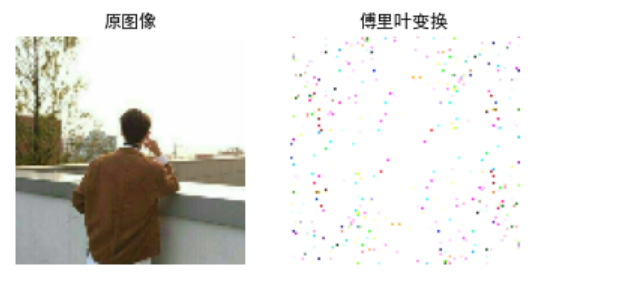上图是将原图进行缩放之后进行傅里叶变换的，只为了降低函数运行时间，尽快的得出结果；当然，OpenCV中也提供了图像傅里叶变换的库函数，接下，我们介绍OpenCV中傅里叶库函数的使用！
3、OpenCV库函数实现傅里叶变换
OpenCV 中相应的函数是cv2.dft()和用Numpy输出的结果一样，但是是双通道的。第一个通道是结果的实数部分，第二个通道是结果的虚数部分，并且输入图像要首先转换成 np.float32 格式。 1）、函数原型：img=cv2.dft(src, flags=None, nonzeroRows=None)
src表示输入图像，需要通过np.float32转换格式flags表示转换标记，其中DFT _INVERSE执行反向一维或二维转换，而不是默认的正向转换；DFT _SCALE表示缩放结果，由阵列元素的数量除以它；DFT _ROWS执行正向或反向变换输入矩阵的每个单独的行，该标志可以同时转换多个矢量，并可用于减少开销以执行3D和更高维度的转换等；DFT _COMPLEX_OUTPUT执行1D或2D实数组的正向转换，这是最快的选择，默认功能；DFT _REAL_OUTPUT执行一维或二维复数阵列的逆变换，结果通常是相同大小的复数数组，但如果输入数组具有共轭复数对称性，则输出为真实数组nonzeroRows表示当参数不为零时，函数假定只有nonzeroRows输入数组的第一行（未设置）或者只有输出数组的第一个（设置）包含非零，因此函数可以处理其余的行更有效率，并节省一些时间；这种技术对计算阵列互相关或使用DFT卷积非常有用
注意，由于输出的频谱结果是一个复数，需要调用cv2.magnitude()函数将傅里叶变换的双通道结果转换为0到255的范围 2）、cv2.magnitude()函数原型：cv2.magnitude(x, y)
x表示浮点型X坐标值，即实部y表示浮点型Y坐标值，即虚部
最终输出结果为幅值，即：

i

m

g

(

I

)

=

x

(

I

)

2

+

y

(

I

)

2

img(I)=\sqrt{x(I)^2+y(I)^2}

3）、傅里叶库函数使用
#傅里叶库函数使用
import cv2
import numpy as np
import matplotlib.pyplot as plt
plt.rcParams['font.sans-serif'] = ['SimHei'] #显示中文
#读取图像
#进行图像裁剪，加快傅里叶运算速率。将原始尺寸缩放为100*100的尺寸
#OpneCV傅里叶变换函数
result =cv2.dft(np.float32(img),flags = cv2.DFT_COMPLEX_OUTPUT)#需要将图像进行一次float转换
#将频谱低频从左上角移动至中心位置
dft_shift = np.fft.fftshift(result)
#频谱图像双通道复数转换为0-255区间
result1 = 20*np.log(cv2.magnitude(dft_shift[:,:,0], dft_shift[:,:,1]))
#图像显示
plt.subplot(121), plt.imshow(img,'gray'), plt.title('原图像')
plt.axis('off')
plt.subplot(122), plt.imshow(result1,'gray'), plt.title('傅里叶变换')
plt.axis('off')
plt.show()使用OpenCV函数需要先将图像转为灰度图，然后才能进行处理，这里林君学长再介绍一个Numpy库的傅里叶变换处理，给出相关代码，小伙伴们自己理解哈，不做说明哦！ 4）、扩展：Numpy库的图像傅里叶变换
#Numpy傅里叶变换
import cv2 as cv
import numpy as np
from matplotlib import pyplot as plt
plt.rcParams['font.sans-serif'] = ['SimHei'] #显示中文
#读取图像灰度图像
#快速傅里叶变换算法得到频率分布
f = np.fft.fft2(img)
#默认结果中心点位置是在左上角,
#调用fftshift()函数转移到中间位置
fshift = np.fft.fftshift(f)
#fft结果是复数, 其绝对值结果是振幅
fimg = np.log(np.abs(fshift))
#展示结果
plt.subplot(121), plt.imshow(img,'gray'), plt.title('原图像')
plt.axis('off')
plt.subplot(122), plt.imshow(fimg,'gray'), plt.title('傅里叶变换')
plt.axis('off')
plt.show()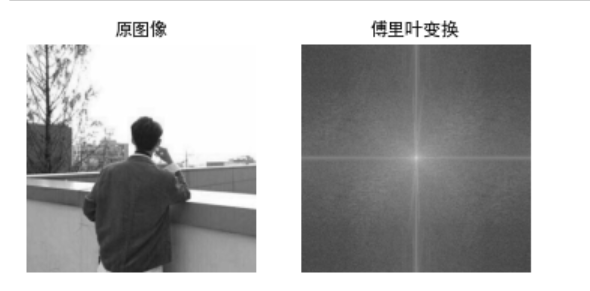二、傅里叶逆变换
1、傅里叶逆变换原理
1）、傅里叶反变换原理就是将图像频域还原为图像时域，具体原理公式如下所示：

f

(

x

,

y

)

=

∑

u

=

0

H

−

1

∑

v

=

0

W

−

1

F

(

u

,

v

)

e

2

j

π

(

u

x

/

H

+

v

y

/

W

)

图

像

高

H

，

宽

W

。

f

(

x

,

y

)

表

示

时

域

图

像

,

F

(

u

,

v

)

表

示

频

域

图

像

。

x

的

范

围

为

[

0

,

H

−

1

]

，

y

的

范

围

为

[

0

,

W

−

1

]

↑

f(x,y)=\sum_{u=0}^{H-1}\sum_{v=0}^{W-1} F(u,v)e^{2j\pi(ux/H+vy/W)} \\\\ 图像高H，宽W。f(x,y)表示时域图像,F(u,v)表示频域图像。x的范围为[0,H-1]，y的范围为[0,W-1] ↑

对于公式的理解，和上面傅里叶变换公式理解一致
2、自定义傅里叶逆变换功能函数
1）、自定义傅里叶逆变换功能函数
import cv2 as cv
import numpy as np
from matplotlib import pyplot as plt
plt.rcParams['font.sans-serif'] = ['SimHei'] #显示中文
#傅里叶反变换
def idft(G):
H, W, channel = G.shape
#定义空白时域图像
out = np.zeros((H, W, channel), dtype=np.float32)
# 准备与原始图像位置相对应的处理索引
x = np.tile(np.arange(W), (H, 1))
y = np.arange(H).repeat(W).reshape(H, -1)
#通过公式遍历
for c in range(channel):
for u in range(H):
for v in range(W):
out[u, v, c] = np.abs(np.sum(G[..., c] * np.exp(2j * np.pi * (x * u / W + y * v / H)))) / np.sqrt(W * H)
# 剪裁
out = np.clip(out, 0, 255)
out = out.astype(np.uint8)
return out

2）、调用函数，先进行傅里叶变换，然后进行傅里叶逆变换并显示结果
#读取图像
#进行图像裁剪，加快傅里叶运算速率。将原始尺寸缩放为100*100的尺寸
img = cv2.resize(img, (100, 100), interpolation=cv2.INTER_CUBIC)
#BGR转换为RGB显示格式，方便通过matplotlib进行图像显示
img=cv2.cvtColor(img,cv2.COLOR_BGR2RGB)
#调用傅里叶变换函数,先将图像进行傅里叶变换，该函数上面我们通过原理写出了哦！
result =dft(img)
#将傅里叶频谱图从左上角移动到中心位置
fshift = np.fft.fftshift(result)
#将复数转为浮点数进行傅里叶频谱图显示
fimg = np.log(np.abs(fshift))
#调用傅里叶逆变换函数
result2=idft(result)
#图像显示
plt.subplot(131), plt.imshow(img), plt.title('原图像')
plt.axis('off')
plt.subplot(132), plt.imshow(fimg), plt.title('傅里叶变换')
plt.axis('off')
plt.subplot(133), plt.imshow(result2), plt.title('傅里叶逆变换')
plt.axis('off')
plt.show()最后我们可以看出，我们通过原理书写的傅里叶变换和傅里叶逆变换是没有问题的，因此，而且傅里叶反变换的结果是和之前的图像一模一样的，几乎没有什么损失，后面我们将会讲到在傅里叶变换的基础上实现高通或者说是低通滤波，来对图像去噪，记得收看啦！
3、OpenCV库函数实现傅里叶逆变换
OpenCV中也提供了傅里叶逆变换的库函数，我们一起看一下函数原型吧 1）、函数原型img=cv2.idft(src,flags,nonzeroRows)
src表示输入图像，包括实数或复数flags表示转换标记nonzeroRows表示要处理的img行数，其余行的内容未定义
2）、OpenCV库函数实现傅里叶逆变换的完整代码如下所示：
#OpenCV实现傅里叶逆变换
import cv2 as cv
import numpy as np
from matplotlib import pyplot as plt
plt.rcParams['font.sans-serif'] = ['SimHei'] #显示中文

#读取图像
#傅里叶变换
dft = cv2.dft(np.float32(img), flags = cv2.DFT_COMPLEX_OUTPUT)#傅里叶变换库函数调用
dftshift = np.fft.fftshift(dft)#将傅里叶频域从左上角移动到中间
res1= 20*np.log(cv2.magnitude(dftshift[:,:,0], dftshift[:,:,1]))#双通道结果转换为0到255的范围用于图像显示
#傅里叶逆变换
ishift = np.fft.ifftshift(dftshift)#将频域从中间移动到左上角
iimg = cv2.idft(ishift)#傅里叶逆变换库函数调用
res2 = cv2.magnitude(iimg[:,:,0], iimg[:,:,1])#双通道结果转换为0到255的范围
#显示图像
plt.subplot(131), plt.imshow(img, 'gray'), plt.title('原图像')
plt.axis('off')
plt.subplot(132), plt.imshow(res1, 'gray'), plt.title('傅里叶变换')
plt.axis('off')
plt.subplot(133), plt.imshow(res2, 'gray'), plt.title('傅里叶逆变换')
plt.axis('off')
plt.show()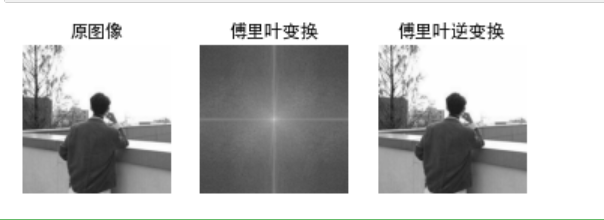3）、扩展：Numpy库也提供图像了傅里叶变换，完整代码如下所示：
#Numpy库的傅里叶变换
import cv2 as cv
import numpy as np
from matplotlib import pyplot as plt
plt.rcParams['font.sans-serif'] = ['SimHei'] #显示中文
#读取图像
#傅里叶变换
f = np.fft.fft2(img) #Numpy的库函数傅里叶变换
fshift = np.fft.fftshift(f)
res = np.log(np.abs(fshift))
#傅里叶逆变换
ishift = np.fft.ifftshift(fshift)
iimg = np.fft.ifft2(ishift) #Numpy的库函数傅里叶逆变换
iimg = np.abs(iimg)#将复数变为实数
#展示结果
plt.subplot(131), plt.imshow(img,'gray'), plt.title('原图像')
plt.axis('off')
plt.subplot(132), plt.imshow(res,'gray'), plt.title('傅里叶变换')
plt.axis('off')
plt.subplot(133), plt.imshow(iimg,'gray'), plt.title('Inverse Fourier Image')
plt.axis('off')
plt.show()以上就是本次博客的全部内容，遇到问题的小伙伴记得留言评论，学长看到会为大家进行解答的，这个学长不太冷！

任何的收获不是巧合，而是每天的努力与坚持得来的。人生因有梦想，而充满动力。 不怕你每天迈一小步，只怕你停滞不前；不怕你每天做一点事，只怕你无所事事。 坚持，是生命的一种毅力！执行，是努力的一种坚持！

陈一月的又一天编程岁月^ _ ^
展开全文计算机视觉 python opencv
• 在检测频率复杂的信号时,光学傅里叶变换的高带宽、快速性优势凸显,其在光谱和高速射频信号的...对两类方案中5种不同系统的输出结果、色散量、光谱分辨率、时间带宽积等影响因素进行分析,分别阐述了其应用及改进方案。
• 也是短时傅里叶变换应用。做的内容是：有一段手机拨号音，用信号处理的办法分析出电话号码是多少。这个东西，当时有个南京大学的大学生吧，好像是，就“听”出里360公司的周老板的电话号码。据说周老板，以及...
由于要带几个本科的同学做点实践性的东西（本科，“数字信号处理”这门课的课程设计），就想了个题目。
标题挺唬人的，还把大教授吓一大跳。以为是要被拉去喝茶的样子……
其实是语谱图的原理与应用。也是短时傅里叶变换与应用。
做的内容是：有一段手机拨号音，用信号处理的办法分析出电话号码是多少。
这个东西，当时有个南京大学的大学生吧，好像是，就“听”出里360公司的周老板的电话号码。据说周老板，以及李开复大老板都向他伸出了橄榄枝。
我这次带的几个小朋友，有2个很认真，能力很强。鼓掌，鼓掌！
以下是项目纲要。我会在后面的一些文章中，谈谈具体怎么实施。

听听黑暗组织BOSS的EMAIL

项目概述
在《名侦探柯南》中，贝尔摩德给“那位先生”发消息时，邮件地址的手机按键音被柯南听见了。拥有绝对音感的他询问了“斯特拉迪瓦里小提琴的不和谐音”案子中的天才音乐家，得知了此声音就是《七个孩子》的旋律。“那位先生”正是黑暗组织的终极BOSS。
尽管我们可能没有江户川同学那样的绝对音感，但是，借助一系列信号处理的手段，我们同样可以从一段拨号音中分析出其对应的电话号码。本项目重点在于基本原理和算法的理解与实现，编程语言为MATLAB，不会用到GUI编程，但鼓励自行钻研。
通过本项目，我们可以进一步理解应用数字信号处理中的一些基本方法和基本原理。例如，傅里叶变换Fourier Transform、快速傅里叶变换Fast Fourier Transform、短时傅里叶变换Short-Time Fourier Transform、时频分析Joint Time-Frequency Analysis等。此外，由于本项目需要进行模式匹配，因此还会初步涉及模式分类。
这些方法不仅有深刻的数学含义与思想方法，也有很大的应用价值。通过本项目，我们可以加深对数字信号处理的理解，也可以初步接触到模式分类，还可以提高自己的编程能力。相信大家在学习相对枯燥的理论知识的同时，可以培养出对这一学科的兴趣。
学以致用。
阶段任务
一、 理论学习阶段
0. 调查项目背景与应用价值。
1. 了解双音多频。
2. 学习几个时频分析的工具，包括短时傅里叶变换、语谱图等。
3. 学习基于频率特征的特征提取。
4. 学习特征向量相似性的度量准则。
二、 编程实现阶段
0. 完成项目方案的整体规划与详细设计。
1. 实现导入手机按键音，并播放。
2. 实现信号的语谱图计算与可视化。
3. 实现信号的频率特征提取与可视化。
4. 实现基于模板的模式分类。
5. 输出电话号码的分析结果。


展开全文语谱图 特征提取 模式匹配
• 离散傅里叶变换（Discrete Fourier Transform，缩写为DFT）指傅里叶变换在时域和频域上都呈现离散的形式，将时域信号的采样变换为在离散时间傅里叶变换（DTFT）频域的采样。 形式上，变换两端（时域和频域）的序列...DFT
• 该系列文章是讲解Python OpenCV图像处理知识，前期主要讲解图像入门、OpenCV基础用法，中期讲解图像处理的各种算法，包括图像锐化算子、图像增强技术、图像分割等，后期结合深度学习研究图像识别、图像分类应用。...
• 上一篇关于电话号码分析的文章，提供了一种实现思路，还放了一些PPT上来。Review：电话拨号声分析之大体...2. 对x整体做傅里叶变换肯定是不行了。只能看见x中所有的频率成分的含量，不能看见什么时候出现的。就会...matlab 特征提取 短时能量
• 关于傅里叶变换的课件，里面详细介绍了傅里叶变换原理方法性质，在工程上的应用等，
• 其实是为了更好地让小朋友们理解傅里叶变换、语谱图，时频分析，等等… 不过，不管怎么样，都要把各个音分出来。 因为录音质量比较好，各个按键之间分得又比较开。所以，用短时能量这个特征足以把各个按键音分开。 ...matlab 特征提取 模式匹配 短时能量
• 听电话拨号音，分析电话号码。第一篇讲了动因，项目纲要。第二篇，把给小朋友们准备的PPT传上来。当然，感谢名侦探柯南。剧场版《战栗的乐谱》里有一段柯南和怜子小姐对着电话听筒合唱110，成功报了警。...matlab 特征提取 短时能量 模式匹配
• 三、傅里叶变换（FT）的引出 四、DTFT、DFT、FFT的引出 第一次认识傅里叶（Fourier）是在大二那年的《信号与系统》课上，当时学这门课也不知道有啥用，听的也是一愣一愣的。。最后也仅仅是达到了期末前三天记了点...数字信号处理
• 一、一些关键概念的引入1、离散傅里叶变换（DFT）离散傅里叶变换(discrete Fourier transform) 傅里叶分析方法是信号分析的最基本方法，傅里叶变换傅里叶分析的核心，通过它把信号从时间域变换到频率域，进而研究...
• 要理解傅里叶变换，我们首先需要了解图像处理。在图像处理的过程中，一般分为空间域处理和频率域处理。 空间域处理是直接对图像内的像素进行处理。空间域处理主要划分为灰度变换和空间滤波两种形式。灰度变换是对...opencv python 图像处理 fft2
• 短时傅里叶变换原理及其MATLAB实现（Short Time Fourier Transform，STFT） 1.短时Fourier变换原理（STFT原理） 信号x(t)短时Fourier变换定义为： 其中w(τ)为窗函数。 X(ω,t)中的时间t表示窗函数w(τ−t)的位置，...
• 张学友演唱会全场就说了一句普通话。“还是要我讲国语啊？” -_-!估计是在问观众，是要我继续说粤语，还是讲普通话 -_-!!反正一句都听不懂 =_=我还从来没听过演唱会。第一次还就是去看这么大牌的。...模式匹配 matlab 特征提取
•  前面转载过一篇关于傅里叶变换原理的文章《一篇难得的关于傅里叶分析的好文》。那篇文章写得非常棒，浅显易懂，可以说稍有基础的人都能看懂那篇博文。但是那篇博文更多的是从信号处理的角度以及原理的角度讲述...图像处理 fft
• 离散傅里叶变换（Discrete Fourier Transform，缩写为DFT）指傅里叶变换在时域和频域上都呈现离散的形式，将时域信号的采样变换为在离散时间傅里叶变换（DTFT）频域的采样。 形式上，变换两端（时域和频域）的序列是...
• 第25章 DSP变换运算-快速傅里叶变换原理（FFT） 在数字信号处理中常常需要用到离散傅立叶变换(DFT)，以获取信号的频域特征。尽管传统的DFT算法能够获取信号频域特征，但是算法计算量大，耗时长，不利于计算机实时对...FFT EMWIN STM32 DFT DSP
• 第25章 DSP变换运算-快速傅里叶变换原理（FFT） 在数字信号处理中常常需要用到离散傅立叶变换(DFT)，以获取信号的频域特征。尽管传统的DFT算法能够获取信号频域特征，但是算法计算量大，耗时长，不利于计算机实时对...DSP EMWIN STM32 FFT DFT
• 文章目录前言傅里叶变换的物理意义附：Matlab代码 前言 记得大学刚开始学...我是从事光电相关行业的，因此接触到大量的傅里叶变换在相关方向上的应用，感叹其带来的便捷。这几篇博文就是想回顾一下傅里叶变换的...
• 前面转载过一篇关于傅里叶变换原理的文章《一篇难得的关于傅里叶分析的好文》。那篇文章写得非常棒，浅显易懂，可以说稍有基础的人都能看懂那篇博文。但是那篇博文更多的是从信号处理的角度以及原理的角度讲述傅里叶...计算机视觉 信号处理
• ## OpenCV中的图像变换——傅里叶变换

万次阅读 多人点赞 2021-07-22 20:11:24
这篇博客将介绍OpenCV中的图像变换，包括用Numpy、OpenCV计算图像的傅里叶变换，以及傅里叶变换的一些应用python opencv
• ## 傅里叶变换(二维离散傅里叶变换)

万次阅读 多人点赞 2018-06-15 22:22:35
离散二维傅里叶变换一常用性质： 可分离性、周期性和共轭对称性、平移性、旋转性质、卷积与相关定理；（1）可分离性： 二维离散傅里叶变换DFT可分离性的基本思想是DFT可分离为两次一维DFT。因此可以用通过计算两次...
• EE261教材， 傅里叶变换及其应用, 从连续时间的傅里叶变换过渡到离散时间的傅里叶变换，并详细讲解了FFT算法， 采样原理等在工程技术中广泛用到的技术。...